### 10 best math solver app with steps by photo 2023

Are you a student or professional looking for maths apps for problem solving? Then you have come to the right place. Here we'll be looking at maths problem solving apps that have been created for students of all ages. While the app format is fantastic for engaging learners on laptops, tablets and mobile devices, it shouldn't be underestimated how useful they can be on desktop systems as well. Let's take a look at some of the best learning apps for helping you develop your numeracy and problem-solving skills.

## 10 Helpful Educational Apps for Maths Problem Solving

When it comes to mathematics problem solving, there are plenty of ways you can utilize certain apps out there. Different apps have different uses, and without the right ones you can get stuck. In this blog post, I'll be reviewing the best maths problem solving apps out there.

## photomath app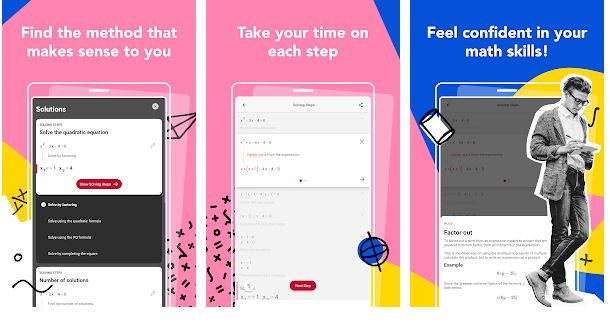Maths problem solving app that explains the steps from problem to solution when tackling maths homework, class assignments and exam questions. Access step-by-step explanations with Maths word problems to help streamline mathematics learning and improve grades. With Photomath's live camera input, simply take a photo of your assignment or textbook question, we will take care of the rest.

PHOTOMATH is a great help for all kinds of students, from those who struggle with math to those who take advanced analysis and everything in between!

## microsoft math solver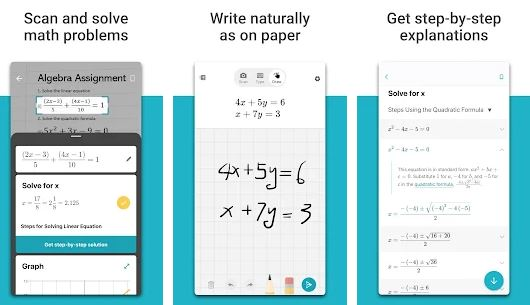Looking for a fancy app to solve math problems? Microsoft Math solver can help you Solve your math problems, just write out the problem on the app or snap a math photo and let Microsoft Math solver do the rest. How does it work? Microsoft Math solver uses artificial intelligence (AI) to understand your problem, initiate step-by-step explanations, and give you relevant examples from millions of problems with similar solutions. It even draws math diagrams with proper notation to solve complex math problems. It’s that simple!

If you want a quick and easy way to solve your math problems, then Microsoft Math Solver app is your best choice! It covers following topics in detail: Arithmetic, Algebra, Trigonometry, Calculus, Statistics, and Many More.

## Maple CalculatorMaple Calculator offers step-by-step solutions for a variety of high school and university-level math topics, including: algebra, graphing, derivatives and much more! Solve math problems of any difficulty level, ranging from basic calculations to university trigonometry or calculus.

The Maple app is available for all skill levels, from basic grade calculations to advanced math puzzles. It’s time to ditch traditional math calculators and use the most reliable math solver on the market.

## mathway algebra calculator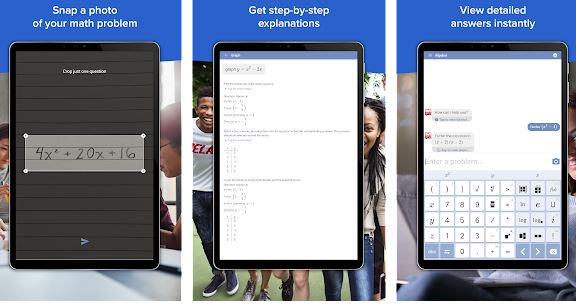Mathway is the world's most intelligent algebra, graphing, calculus and other mathematical calculators! Just point the camera at and take a photo or type in your math homework question to get a step-by-step answer.

Mathway is a unique math problem solving app which help you solve any math problem. mathematics calculator can assist you in matters of algebra, geometry, trigonometry, calculus, pre algebra and so much more.

## symbolab math solver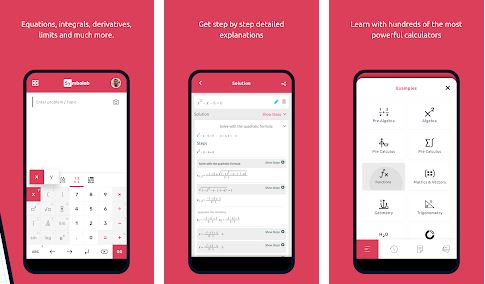The Symbolab Photo Math Answer & Scanner is here to help you solve any math problem with step-by-step instructions. It works just like a real math tutor. Every time you are stuck, take a picture of your problem and the app will tell you exactly what to do!

With Symbolab you have the world's most advanced math photo calculator and scanner built directly into your smartphone. Over 200 millions students in more than 70 countries have trusted Symbolab to help them work through and understand their toughest math problems, earning a perfect 4.9/5 rating from users.

## cymath math problem solver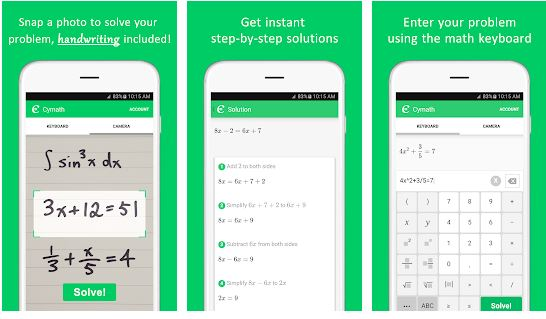Cymath math app you can solve your entire math homework or learn algebra and calculus step by step. You can even scan the QR code with your mobile phone or share the math problem on Facebook and Twitter to get help from your friends.

Cymath's advanced math system solves your problem automatically and provides step-by-step solutions giving you the know-how to answer any fresh or old math homework problem. their algebra solver answers all types of math problems and provides the steps to solving each question.

## Camera Math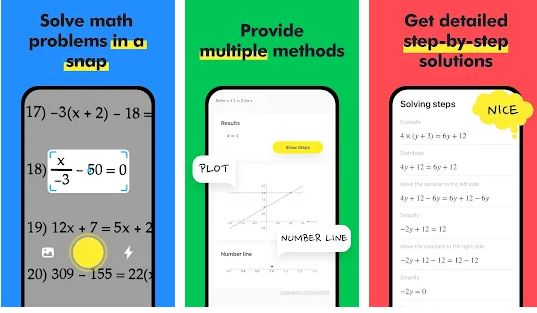Camera Math is an application designed to help you solve math problems, or to learn better by yourself. And it doesn’t matter whether you’re a student or not. Anyone who wants to improve their proficiency in mathematics can use this application.

Camera Math snaps a picture of any algebraic expressions and delivers answers immediately, also it provides step-by-step explanations for each problem. Get immediate solutions for math equations with help from Camera Math.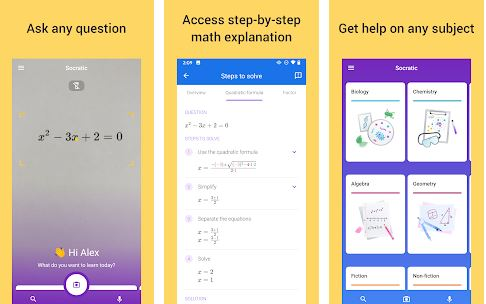Socratic by Google is all about problem solving. As a math tutor app, Socratic understands the concepts that you don’t, and it finds the best online resources to help you understand them. You ask questions and Socratic finds answers.

Socratic is an AI-powered tool that teaches maths. This math app can help you get unstuck on topics like algebra, equations and more.

## mathpapa equation solver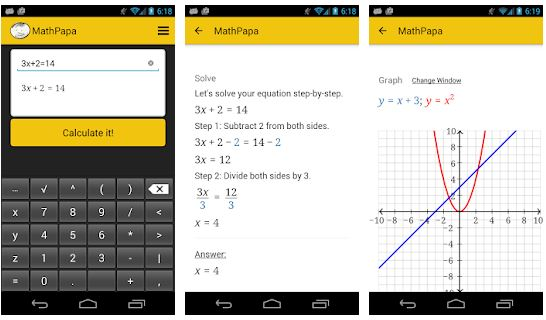MathPapa is a math problem solving app that lets you graph, factor, simplify and find step-by-step solutions to your algebra math problems. It works off-line on your iOS and android devices so you can use it anywhere.just enter your question, and MathPapa will show you the solution to your math work.

## Calculus Problem Solver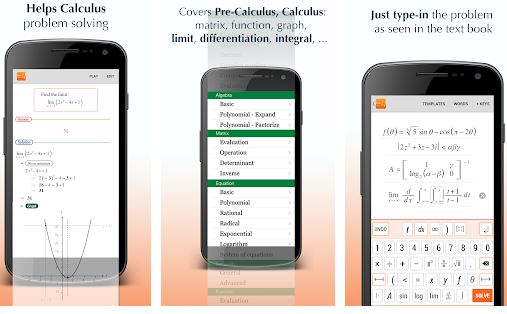Are you facing difficulties with your college math homework? Are your exams getting closer and you have still not finished your math problems? Or perhaps you are having a hard time even starting? If the answer is yes, then this might be exactly the app to help you.

It's an advanced calculus problem solver app with an extensive database of math problems that are academic to the school grade. It has been proven that students have higher pass rates in math exams and courses by using the solver.

This best math solver app with steps by photo will be a useful tool for students of all grade levels and skill sets. Whether they are looking to find the derivative or intersecting lines, students and teachers alike will benefit from the easy interface and step by step problem solving process.